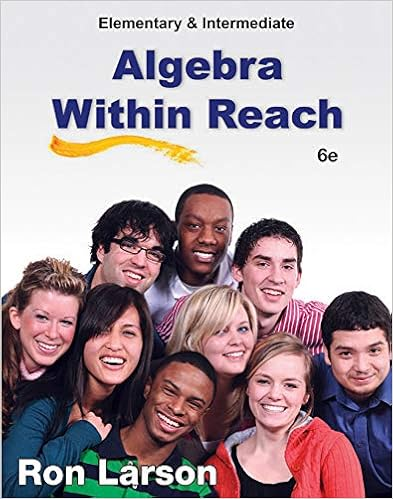# 104 1 1 2 3 4 5 6 7 8 1 1 2 3 4 5 we can use 0 0 as a

• Notes
• 124
• 100% (2) 2 out of 2 people found this document helpful

This preview shows page 104 - 111 out of 124 pages.

##### We have textbook solutions for you!
The document you are viewing contains questions related to this textbook.The document you are viewing contains questions related to this textbook.
Chapter 3 / Exercise 23
Elementary and Intermediate Algebra: Algebra Within Reach
LarsonExpert Verified
104
##### We have textbook solutions for you!
The document you are viewing contains questions related to this textbook.The document you are viewing contains questions related to this textbook.
Chapter 3 / Exercise 23
Elementary and Intermediate Algebra: Algebra Within Reach
LarsonExpert Verified
-1012345678-1012345We can use(0,0)as a test point: it makesthe inequality true, so we shade the lowerleft half-plane.The point(0,0)is the easiest test point to use, but remember that you can use it only if line doesnotpass through the origin.Inequality I:-1012345678-1012345Inequalities I and II together:-1012345678-1012345Final Answer:105
-1012345678-1012345The final answer is the region that satisfiesallthe inequalities from the original system.There are two methods for putting the separate inequalities together.1. Use a different color for each—then the final answer is the darkest region;2. use each inequality to successively eliminate part of the plane—the the final answer is what is leftover.Here’s the last problem done using successive elimination.Inequality I (red means eliminated):-1012345678-1012345Inequality II (the final answer is white):106
-1012345678-1012345We’re interested in using these graphs to solve LP problemsso our systems will usually include thenatural constraints.Example:x0y0x+ 2y4Iy7-3xIIThe addition of the natural constraints produces aslightchange:sincexandymust both benonnegative,the answer includes only points from the first quadrant.-1012345678-1012345IIIIn the context of LP problems, this is called thefeasible set.We need to know a little bit more about the feasible set:We also need to know the coordinates ofthe corners.107
-101234-101234IIIABCDAis obviously(0,0).BandDare also easy:they’re intercepts of the lines.-101234-101234IIIABCDBis thex-interceptof II:y= 7-3x.So0=7-3x7/3=x,andB= (7/3,0).Similarly,Dis they-intercept of I:x+ 2y= 4.0 + 2y=4y=2D=(0,2)-101234-101234IIIABCDCis the only hard one.It is formed by the intersection of lines Iand II, so we must solve a system of linearequations to find it:x+ 2y=4y=7-3x.108
You can use any method you like to solve the system. This one is primed for the substitution methodbecause equation By substitution,x+ 2(7-3x)=4-5x+ 14=4-5x=-10x=2y=7-3(2) = 1.SoC= (2,1). Now we have all the corners.A(0,0)B(7/3,0)C(2,1)D(0,2)This table will be crucial in actually solving LP problems.7.1Solving LP ProblemsNow suppose our objective is to maximize the value of3x+ 2yon the feasible set. Here is the previousfeasible set together with the values of3x+ 2yindicated by color: green for high values and red for lowvalues.Of all the points in the feasible set, whichis the greenest?Suppose we were looking for the mini-mum?Now suppose the objective function isx-y. Remember: green for high values and red for low values.Of all the points in the feasible set, whichis the greenest?Which is the reddest?109
It seems that both the max and min values are at corner points.In fact this always trueif theobjective function is linear.With a linear objective function,the color gradient is straight.
•••